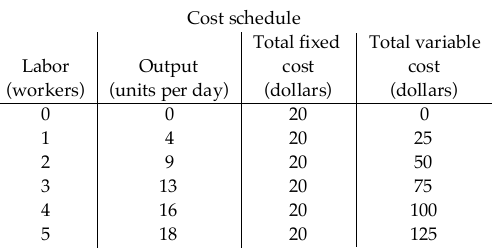Bài sắp đăng
Home | Trắc nghiệm | Kinh tế học | Trắc nghiệm Kinh tế Vi mô – C10 (EN)Ngân hàng câu hỏi trắc nghiệm Kinh tế Vi mô

# Trắc nghiệm Kinh tế Vi mô – C10 (EN)

1. The short run is a period of time in which
A. nothing the firm does can be altered.
B. the amount of output is fixed.
C. prices and wages are fixed.
D. the quantities of some resources the firm uses are fixed.

Correct: D

2. The short run is a period of time in which
A. output prices are fixed.
B. the quantity used of at least one resource is fixed.
C. resource prices are fixed.
D. the quantities used of all resource are fixed.

Correct: B

3. The short run is a time frame in which
A. the quantities of some resources are fixed and the quantities of other resources can be varied.
B. the quantities of all resources are fixed.
C. the quantities of all resources can be varied.
D. all costs are sunk costs.

Correct: A

4. An example of a variable resource in the short run is
A. an employee.
B. capital equipment.
C. land.
D. a building.

Correct: A

5. A cost that has already been made and cannot be recovered is called a
A. marginal cost.
B. fixed cost.
C. variable cost.
D. sunk cost.

Correct: D

6. The long run is a time frame in which
A. the quantities of all resources are fixed.
B. the quantities of all resources can be varied.
C. the quantities of some resources are fixed and the quantities of other resources can be varied.
D. all costs are sunk costs.

Correct: B

7. In the long run, a firm can vary
A. its capital but not its labor.
B. its labor but not its capital.
C. both its labor and its capital.
D. neither its labor nor its capital.

Correct: C

8. The long run is distinguished from the short run in that, in the long run,
A. output prices can vary.
B. the firm no longer maximizes its profit.
C. resource prices can vary.
D. the quantities of all resources can be varied.

Correct: D

9. The marginal product of labor is the increase in total product from a
A. one dollar increase in the wage rate, while holding the price of capital constant.
B. one unit increase in the quantity of labor, while also increasing the quantity of capital by one unit.
C. one unit increase in the quantity of labor, while holding the quantity of capital constant.
D. one percent increase in the wage rate, while also increasing the price of capital by one percent.

Correct: C

10. The marginal product of labor is the change in total product from a one-unit increase in
A. the wage rate.
B. both the quantity of labor and the quantity of capital employed.
C. the quantity of labor employed, holding the quantity of capital constant.
D. the quantity of capital employed, holding the quantity of labor constant.

Correct: C

11. The marginal product of labor is the
A. output level above which the slope of the total product curve falls.
B. output level above which the rate of total product per unit of labor falls.
C. maximum output attainable with fixed factors when labor is the only variable factor.
D. change in output resulting from a one-unit increase in labor.

Correct: D

12. The average product of labor is
A. the inverse of the average product of capital.
B. total product divided by the total quantity of labor employed.
C. the slope of the curve showing the total product of labor.
D. the slope of the curve showing the marginal product of labor.

Correct: B

13. Average product is the
A. maximum output attainable with fixed factors and one variable factor.
B. total product per unit of an input.
C. change in total product due to a one unit change in input.
D. total product divided by the total cost.

Correct: B14. In the above table, the total product that is produced when the firm employs four workers is
A. 8
B. 15
C. 3.75
D. 3

Correct: B

15. In the above table, the marginal product of the third worker is
A. 2.
B. 1.
C. 4.
D. 3.

Correct: C

16. In the above table, the marginal product of the fourth worker is
A. 6.
B. 3.
C. 1.
D. 4.

Correct: B

17. In the above table, the marginal product is greatest when the
A. third worker is hired.
B. second worker is hired.
C. fourth worker is hired.
D. first worker is hired.

Correct: B

18. In the above table, the average product of three workers is
A. 1.
B. 4.
C. 3.
D. 2.

Correct: B

19. In the above table, the average product is less than the marginal product
A. when the first worker is hired.
B. when the second worker is hired.
C. for the entire range of output given.
D. when the third worker is hired.

Correct: B

20. Points below a firm’s total product curve are
A. technologically efficient but not attainable.
B. both attainable and technologically efficient.
C. attainable but not technologically efficient.
D. neither attainable nor technologically efficient.

Correct: C